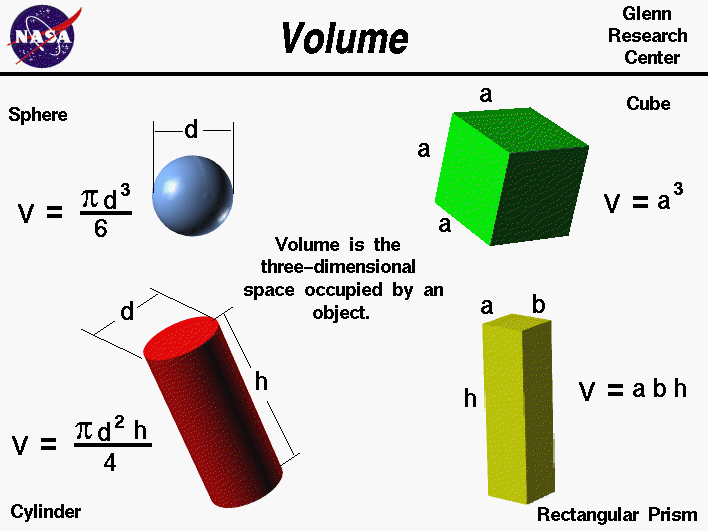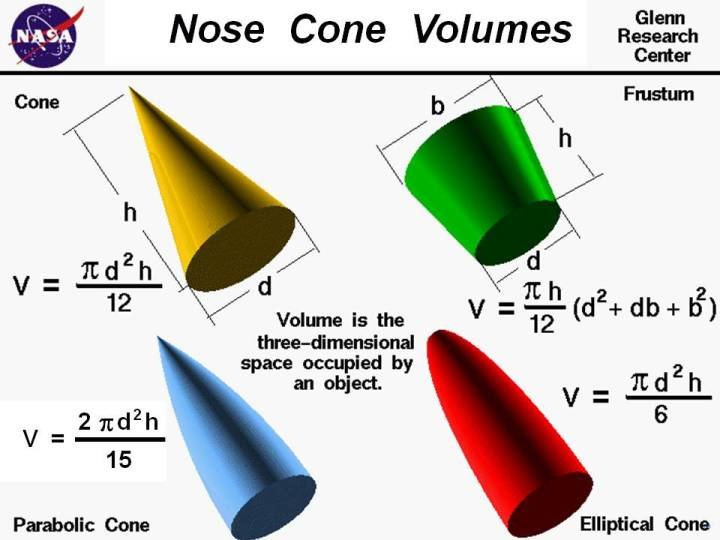+ Text Only Site
+ Non-Flash Version
+ Contact GlennOne can calculate the weight of any object by multiplying the density of the material by the volume of the object. The volume of an object is the three-dimensional space that is occupied by the object and we are taught formulas for computing the volume of some simple three-dimensional objects in middle school. On this slide, we list some equations for computing the volume of objects which often occur in aerospace. There are similar equations for computing the area of objects. The magnitude of the aerodynamic forces depends on the surface area of an object, while the gravitational force and certain thermodynamic effects depend on the volume of the object. The equations to compute area and volume are used every day by design engineers. A simple check on any formula for area or volume is a dimensional check. Area is the two-dimensional amount of space that an object occupies. Area is measured along the surface of an object and has dimensions of length squared; for example, square feet of material, or square centimeters. Volume is the three-dimensional amount of space that an object occupies. Volume has dimensions of length cubed; for example, cubic feet of material, or cubic centimeters (cc's). For a sphere, the distance from one point on the surface to another point on the surface measured through the center of the sphere is called the diameter. The volume V of a sphere is equal to pi (3.14159) times the diameter d cubed divided by six; V = pi * d^3 / 6 Since the diameter is twice the radius r of the sphere, this equation can also be written as: V = pi * 4 * r^3 / 3 For a circular cylinder, we need to know the length h of the cylinder and the diameter d of the circular cross section. Then the equation for the volume is pi times the diameter squared times the length divided by four; V = pi * d^2 * h / 4 For a rectangular prism with dimensions a, b, and h the volume is the product of the three lengths; V = a * b * h A cube is a special case of a rectangular prism in which all the sides are equal to a. The volume equation then becomes; V = a^3 Here are some special volume equations which are used for rocket nose cones:For a cone, the distance from the tip to the base is called the height. The base is a circle of diameter d. The volume V of a cone is equal to pi (3.14159) times the diameter d squared times the height h divided by twelve; V = pi * d^2 * h / 12 A parabolic cone has a smooth curved surface and a sharp pointed nose. On the standard cone there is an edge between the nose and the cylinder which forms the body of the rocket. But on the parabolic cone, the surface comes into the base with a slope equal to zero. There is no edge between the parabolic nose cone and the cylindrical rocket body . The equation for the volume is two times pi times the diameter d squared times the height h divided by fifteen; V = 2.0 * pi * d^2 * h / 15 An elliptical cone is similar to the parabolic cone except the nose is blunted and not sharp. If the nose cone were cut in half, perpendicular to the base, the resulting cross-section would be half of an ellipse. The equation for the volume is pi times the diameter d squared times the height h divided by six; V = pi * d^2 * h / 6 The frustum of a cone is formed if the tip is cut off parallel to the base. Frustum shapes occur often on model rockets as fairings between cylindrical sections of the body. The equation for the volume is pi times the height h divided by twelve times the quantity: base diameter b squared plus base diameter times cut diameter d plus cut diameter squared: V = (pi * h / 12) * (d^2 + d*b + b^2) Notice: On all of the shapes that have curved (circular) surfaces, we are using the diameter of the circle in the derivation of the volume. We are not using the radius that is often used in mathematics text books. The reason for this choice is that most standard engineering measurements are based on diameter, not radius. It is easier to accurately measure the diameter of a circular object than to measure the radius. To use the radius, you need to determine where the center of the circle lies. For a tube, there is no material along the center of the circle. For a sphere, the center cannot be reached since it is inside the body. You can convert from diameter to radius (radius = diameter / 2). Activities: Guided Tours Navigation ..Beginner's Guide Home Page+ Inspector General Hotline + Equal Employment Opportunity Data Posted Pursuant to the No Fear Act + Budgets, Strategic Plans and Accountability Reports + Freedom of Information Act + The President's Management Agenda + NASA Privacy Statement, Disclaimer, and Accessibility CertificationEditor: Nancy Hall NASA Official: Nancy Hall Last Updated: May 07 2021 + Contact Glenn